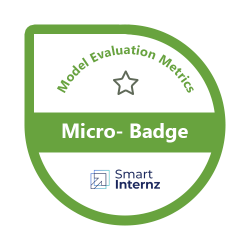##### Foundational
• Duration 4 Hrs
• Cost Free
• Enrollments 180
• Tech Stack Python
• Project(s) 1## Model Evaluation Metrics

This badge earner can evaluate the performance of machine learning models developed with Regression and Classification algorithms. Earning this badge will help you to learn following evaluation metrics
• Confusion matrix
• Accuracy
• Precision
• Recall
• Specificity
• F1 score
• Precision-Recall or PR curve
• ROC (Receiver Operating Characteristics) curve
• PR vs ROC curve.

##### Skills you Gain
Python Machine Learning Regression Algorithms Classification Algorithms Regression Algorithms
##### Projects(s)

Evaluation of Regression & Classification Models

##### Benefits
Build expertise in Machine Learning